How FEA on 3D magnetics works

SMEKlib goes 3D!

…with baby steps.

Sidenote: is that expression supposed to mean step-babies, i.e. the offspring of Ma and Pa Step, or the steps that babies take. Because if it is the latter, it doesn’t make any sense. Babies don’t take steps – toddlers do!

To be exact, you can now download and run a small example from GitHub. (Note: the link directs to the work-in-progress branch, the only one with the example in the moment of writing. In case you are reading this in the future and the link doesn’t work, just follow the default link on the top of this page).

The problem consists of a unit cube – all edges 1 m – with a rectangular conductor carrying a fixed current to the z-direction. The resulting magnetic field is then solved and visualized.

At the moment, there are four files:

busbar.geo is an input file for the gmsh meshing software. The bottom of the square is first defined as a 2D geometry, and then extruded to form the cube. Normally, you don’t have to touch this file, unless you want to change the mesh density or the geometry.

busbar.msh is the resulting mesh created by gmsh. This you shouldn’t touch, other than by overwriting it with another gmsh output if need be.

load_mesh.m loads the abovementioned mesh into Matlab. The functionality will probably be moved into the gwrap wrapper class later on.

example_solve.m finally solves the problem. There is an option to use a direct matrix solver, or an iterative one. The former approach is fine for smaller problems, but it will quickly run out of RAM if the mesh density is increased. Thus, it’s commented away by default.

### How it works

So, what is solved, exactly? Well, remember the post-it version of FEA? We start from the integral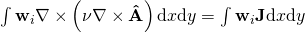for all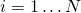,

but don’t use it as such. The reason for this is the nasty curl-curl double differentiation, demanding too much from our shape functions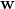. To get rid of it, we first apply the magic identity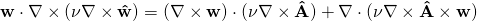The firs part (curl-curl) is fine-to-integrate as such, while the second one can be transformed into a boundary integral by using the Gauss theorem: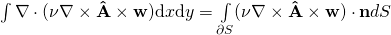.

Finally, after a little more triple product magic, the boundary integral is transformed into something like (=I may have gotten the order and/or signs wrong…)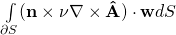.

Now, we see that the term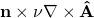is indeed the tangential component of the magnetic field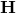on the outer boundary (as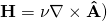. If we know it, we can simply plug it into the integral, and it’s taken care automatically. Often, it is set to zero, in which case the field will be perpendicular to the boundary.

In the example, however, we have a different boundary condition called a Dirichlet condition. Indeed, we have no flux leaving the box, so we want the normal component of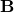to be zero. This would mean that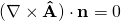,

but in practice this is achieved by just setting the tangential component of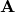to zero on the boundary.

##### Setting the Dirichlet condition

Now, remember that our solution is approximated as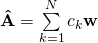.

In our example, the functionsare something called Nedelec shape functions (of the lowest order from the first family, to be exact), also called Nedelec edge elements. As their name suggests, we have one function per edge – a line connecting two nodes. Furthermore, the functions are such that they have a tangential component on only those faces (the outer triangular surfaces making up each tetrahedral element) that share the particular edge.

Now, setting up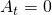on the outer boundary can be accomplished by simply selecting those edges – those shape functions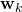on the boundary – and setting the corresponding coefficients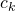to zero. This is exactly what is done in the example.

### Solvability

There’s another issue to tackle, too. As the vector potential is defined “backwards” by the flux density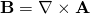,

it is not unique. Hence, the problem isn’t straightforward to solve with a computer.

This happens because we can add any gradient toand still get the same. One way to ensure a unique solution would be to enforce a so-called gauge condition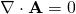(see here for one way how to do this). However, there’s also a simpler, albeit ugly way, to do this.

Because of mathematics, the gauge condition is not needed for eddy-current problems: problems with electrically conductive materials and time-varying fields. Mathematically, the FEA problem then looks like this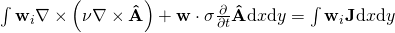for all,

where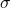is the conductivity and the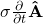the induced current density, correspondingly.

So, the quick-and-dirty way of solving the solvability problem is to add a little conductivity everywhere, by setting the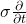term to a small value.

Of course, the solution won’t be exactly correct after this. It’ll get better whenis reduced, but the problem will get harder for the computer at the same time.

##### Iterative solution

Another option is to use an iterative solver. The conjugate gradient method is the textbook example, but unfortunately won’t often work on (the nonsymmetric and indefinite) magnetics problems. Therefore, I prefer to use either the biconjugate version, or gmres.

Iterative methods are nice in the sense that they don’t care if the solution is unique or not. They simply return some solution that fulfills the original field problem.

Unfortunately, there are a few catches. The methods rarely work as such, but instead require preconditioning. For that purpose, the incomplete LU factorization (ILU) is a common black-box approach.

Furthermore, it may generally happen that the fixed current density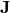does not play well with the Nedelec elements. To fix this part, we can for instance replaceby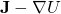, where the gradient field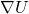is solved in advance to satisfy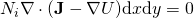for all nodal shape functions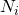. But I digress.

At the moment, the SMEKlib example does not have this part implemented, but seems to work alright nevertheless. However, this is probably due to the very simple geometry, and I suspect that having any curvature in the current density would complicate things a lot.

### Conclusion

• SMEKlib now has a little example on 3D magnetics.
• You now understand 3D magnetics.
• Here is an artistic masterpiece, demonstrating the dependency between the magnetic field and the current density.